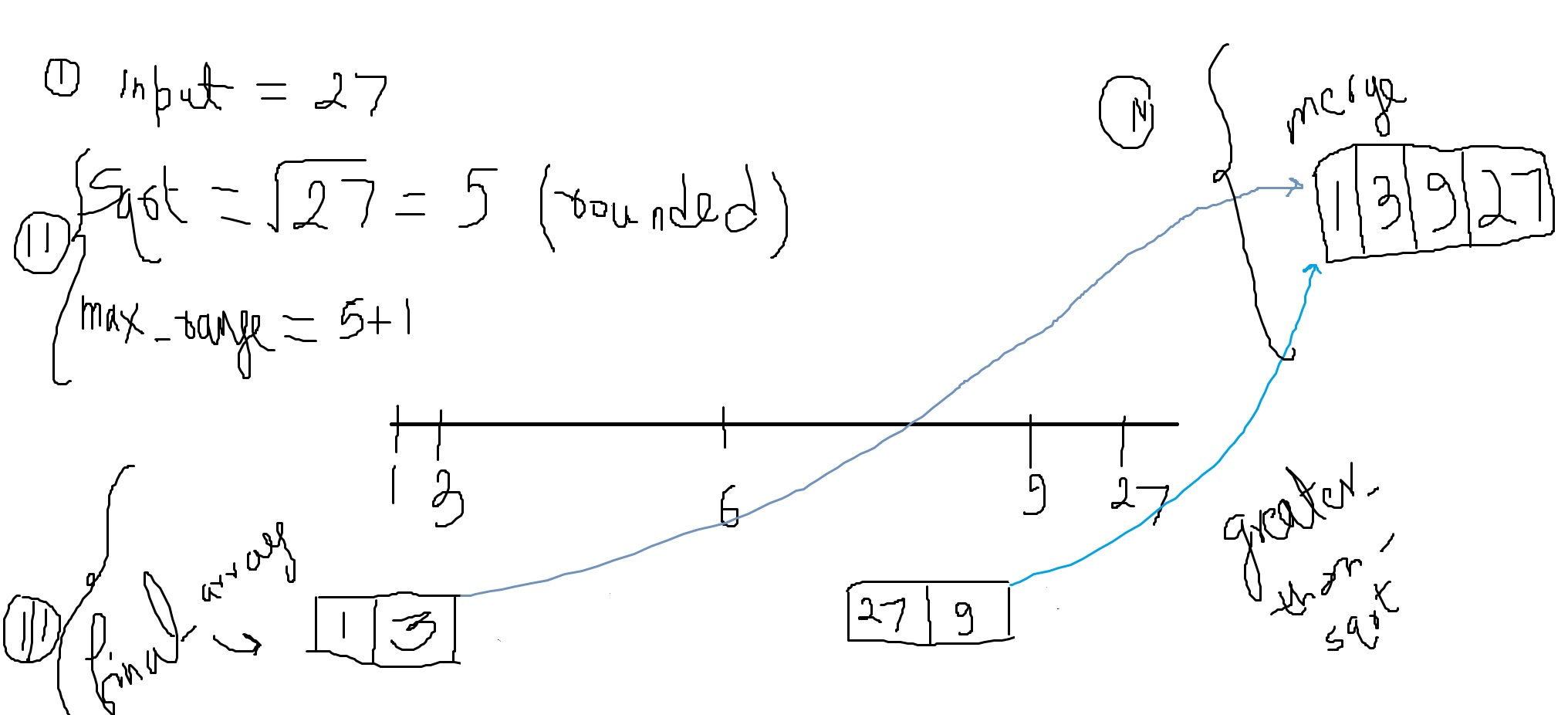# CLEAN CODE: ALL FACTORS OF A NUMBER

By: Saurav

2017-11-11 04:55:00 UTC

Given a number N, find all factors of N.

Example:

N = 6
factors = {1, 2, 3, 6}
Make sure the returned array is sorted.

InterviewBit

This is a simple question where you can just iterate through 1 to that given number and print out those number which divides it. And that does solve the problem.

But in an interview, by asking this question the interviewer wants to see if you can work up a nice efficient solution.

To get started, lets start by writing few tests:

```p find_factors_of_number(1) == 
p find_factors_of_number(2) == [1, 2]
p find_factors_of_number(3) == [1, 3]
p find_factors_of_number(18) == [1, 2, 3, 6, 9, 18]
p find_factors_of_number(27) == [1, 3, 9, 27]
p find_factors_of_number(30) == [1, 2, 3, 5, 6, 10, 15, 30]
p find_factors_of_number(36) == [1, 2, 3, 4, 6, 9, 12, 18, 36]```

Attempt 1:

```  def find_factors_of_number(input_number)
return [input_number] if input_number < 2
return [1, input_number] if input_number < 3

final_array, greater_than_sqrt = Array.new, Array.new
(1..Math.sqrt(input_number)).each do |i|
if input_number%i == 0
final_array << i
greater_than_sqrt << input_number/i
end
end

return merge_lesser_and_greater_arrays(final_array, greater_than_sqrt)
end

def merge_lesser_and_greater_arrays(final_array, greater_than_sqrt)
(greater_than_sqrt.length-1).downto(0).each do |i|
final_array << greater_than_sqrt[i]
end
return final_array.uniq
end```

Lets make it a bit cleaner and remove extra actions taken by one function. So each function does one thing and just one thing best.

Attempt 2:

```def find_factors_of_number(input_number, final_array = Array.new, greater_than_sqrt = Array.new)
return return_appropriate_factors_if_smaller_than_four(input_number) if input_number < 4

(1..Math.sqrt(input_number)+1).each do |i|
update_each_arrays(final_array, greater_than_sqrt, i, input_number) if input_number%i == 0
end

return merge_lesser_and_greater_arrays(final_array, greater_than_sqrt)
end

def update_each_arrays(final_array, greater_than_sqrt, i, input_number)
final_array << i
greater_than_sqrt << input_number/i
end

def merge_lesser_and_greater_arrays(final_array, greater_than_sqrt)
(greater_than_sqrt.length-1).downto(0).each do |i|
final_array << greater_than_sqrt[i]
end
return final_array.uniq
end

def return_appropriate_factors_if_smaller_than_four(input_number)
return [input_number] if input_number < 2
return [1, input_number] if input_number < 4
end
```All our tests pass and we have a much efficient solution.

Time complexity: (O(sqrt(n)) + O(k)), where k would be the number of factors

If we add all elements in only one array and then decide to sort it, our complexity will be: (O(sqrt(n)) + O(klogk)) which is greater than what we are using.

In the figure below, I have tried to explain the algorithm I used:

Step 1: Get square root of the number
Step 2: Build two arrays, one stores smaller than the square root and the other stores greater than the square root
Step 3: Iterate though 1 till the (square root+1) and add the perfect divisor and its remainder in smaller and greater array
Step 4: Iterate the greater array in reverse order, adding smaller elements to the smaller array first.
Step 5: Return the uniq of the merged array### Let me know what you think! twitter: sprakash24octlinkedin

Owned & Maintained by Saurav Prakash

If you like what you see, you can help me cover server costs or buy me a cup of coffee though donation :)